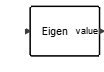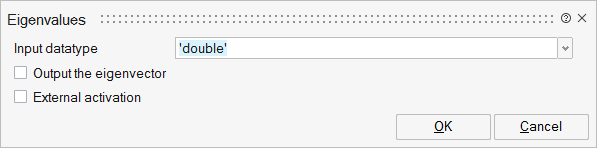# Eigenvalues

This block computes the eigenvalues and the eigenvectors of a square input matrix U. The output is a vector containing the eigenvalues. Optionally, the outputs could be a diagonal matrix composed of the eigenvalues and a matrix composed of the eingenvectors represented by its columns.MatrixOperations

## Description

The Eigenvalues block computes the eigenvalues and the eigenvectors of a square input matrix U.

By default, the block outputs the eigenvalues in a vector form; if the input is a M-by-M matrix the output is a M-by-1 vector.

If the output of the eigenvector parameter is turned on, the block outputs two matrices: the first output is an M-by-M diagonal matrix composed of the eigenvalues, and the second is an M-by-M matrix composed of the eigenvectors; the eigenvectors are represented by the columns of the matrix.

## ParametersNameLabelDescriptionData TypeValid Values

typ

Input datatype

It indicates the input data type. It can be: double, complex or inherit.

String

'double'
'complex'
'inherit'

decomptyp

Output the eigenvector

If selected, the block computes both eigenvalues and eigenvectors, otherwise only the eigenvalues are computed.

Number

0
1

externalActivation

External activation

Specifies whether the block receives an external activation or inherits its activation through its regular input ports. When External Activation is selected, an additional activation port is added to the block. By default, external activation is not selected.

Number

0
1

## Ports

NameTypeDescriptionIO TypeNumber

Port 1

explicit

input

1

value

explicit

output

1

Port 3

explicit

output

decomptyp

Port 4

activation

input

externalActivation

NameValueDescription

always active

no

direct-feedthrough

yes

zero-crossing

no

mode

no

continuous-time state

no

discrete-time state

no